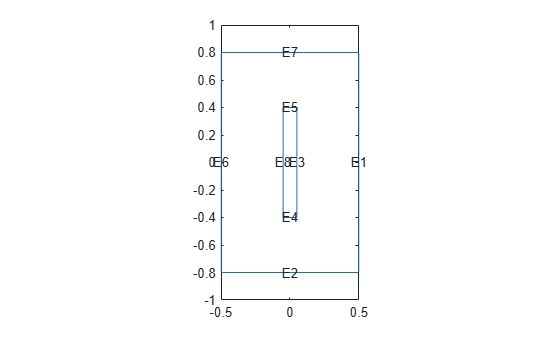# linearizeOutput

Specify outputs of linearized model

## Syntax

``linearizeOutput(model,RegionType,RegionID)``
``linearizeOutput(model,RegionType,RegionID,'Component',xyz)``
``output = linearizeOutput(___)``

## Description

example

````linearizeOutput(model,RegionType,RegionID)` adds all degrees of freedom (DoFs) associated with the region defined by `RegionType` and `RegionID` to the output vector of the linearized model. For 3-D structural models, `linearizeOutput` adds all x-coordinates first, then all y-coordinates, then all z-coordinates. In the linearized model `sys`, use `sys.OutputGroup` to locate the sections associated with each coordinate.Use `linearizeInput` and `linearizeOutput` together with the `linearize` function to extract sparse linear models from structural and thermal models.```

example

````linearizeOutput(model,RegionType,RegionID,'Component',xyz)` specifies which of the coordinates to include.```
````output = linearizeOutput(___)` returns a structure array `output` with the linearization output description. Use this syntax with any of the previous arguments.```

## Examples

collapse all

Specify the regions of a 2-D thermal model for which `linearize` extracts sparse linear models used in Control System Toolbox™.

Create a transient thermal model.

`thermalmodel = createpde('thermal','transient');`

Add the block geometry to the thermal model by using the `geometryFromEdges` function. The geometry description file for this problem is called `crackg.m`.

`geometryFromEdges(thermalmodel,@crackg);`

Plot the geometry, displaying edge labels.

```pdegplot(thermalmodel,'EdgeLabels','on') ylim([-1,1]) axis equal```Generate a mesh.

`generateMesh(thermalmodel);`

Specify the thermal conductivity, mass density, and specific heat of the material.

```thermalProperties(thermalmodel,'ThermalConductivity',1, ... 'MassDensity',1, ... 'SpecificHeat',1); ```

Specify the temperature on the left edge as `100`, and constant heat flow to the exterior through the right edge as `-10`. Add a unique label to each boundary condition.

```thermalBC(thermalmodel,'Edge',6,'Temperature',100,'Label','TempBC'); thermalBC(thermalmodel,'Edge',1,'HeatFlux',-10,'Label','FluxBC');```

Specify that the entire geometry generates heat and add a unique label to this assignment.

`internalHeatSource(thermalmodel,25,'Label','HeatSource');`

Set an initial value of `0` for the temperature.

`thermalIC(thermalmodel,0);`

Call the `linearizeInput` function with the previously defined labels for the boundary conditions and the internal heat source to set the inputs for the `linearize` function. Add one label per function call.

```linearizeInput(thermalmodel,'HeatSource'); linearizeInput(thermalmodel,'TempBC'); linearizeInput(thermalmodel,'FluxBC');```

Call the `linearizeOutput` function to specify the regions for which you want `linearize` to extract sparse linear models. Specify one region per function call.

`linearizeOutput(thermalmodel,'Edge',2)`
```ans = struct with fields: RegionType: 'Edge' RegionID: 2 ```

Specify which of the x-, y-, and z- coordinates to include in a linearized model.

Create a structural transient analysis model.

`structuralmodel = createpde('structural','transient-solid');`

Import and plot the tuning fork geometry.

```importGeometry(structuralmodel,'TuningFork.stl'); pdegplot(structuralmodel)```Generate a mesh.

`generateMesh(structuralmodel,'Hmax',0.005);`

Specify Young's modulus, Poisson's ratio, and the mass density to model linear elastic material behavior. Specify all physical properties in consistent units.

```structuralProperties(structuralmodel,'YoungsModulus',210E9, ... 'PoissonsRatio',0.3, ... 'MassDensity',8000);```

Identify faces for applying boundary constraints and loads by plotting the geometry with the face labels.

```figure('units','normalized','outerposition',[0 0 1 1]) pdegplot(structuralmodel,'FaceLabels','on') view(-50,15) title 'Geometry with Face Labels'```Impose sufficient boundary constraints to prevent rigid body motion under applied loading. Typically, you hold a tuning fork by hand or mount it on a table. As a simplified approximation of this boundary condition, fix a region near the intersection of the tines and the handle (faces 21 and 22).

`structuralBC(structuralmodel,'Face',[21,22],'Constraint','fixed');`

Specify the pressure loading on a tine as a short rectangular pressure pulse.

```structuralBoundaryLoad(structuralmodel,'Face',11,... 'Pressure',5E6, ... 'EndTime',1e-3, ... 'Label','Pressure');```

Specify acceleration due to gravity as a body load.

```structuralBodyLoad(structuralmodel, ... 'GravitationalAcceleration',[0 0 -1], ... 'Label','Gravity');```

Create inputs for gravity and the pressure pulse on tuning fork.

```linearizeInput(structuralmodel,'Gravity'); linearizeInput(structuralmodel,'Pressure');```

Measure the y-displacement of face 12 and x-displacement of face 6.

`linearizeOutput(structuralmodel,'Face',12,'Component','y')`
```ans = struct with fields: RegionType: 'Face' RegionID: 12 Component: 'y' ```
`linearizeOutput(structuralmodel,'Face',6,'Component','x')`
```ans = struct with fields: RegionType: 'Face' RegionID: 6 Component: 'x' ```

## Input Arguments

collapse all

Structural or linear thermal model, specified as a `StructuralModel` object or a `ThermalModel` object. The `linearize` function does not support nonlinear thermal analysis.

Geometric region type, specified as `'Cell'` (for a 3-D model only), `'Face'`, `'Edge'`, or `'Vertex'`.

Data Types: `char`

Geometric region ID, specified as a positive integer. Find the region IDs by using `pdegplot` with the `'CellLabels'`, `'FaceLabels'`, `'EdgeLabels'`, or `'VertexLabels'` value set to `'on'`.

Data Types: `double`

Coordinates to include, specified as a character vector or a string of x-, y-, and z-coordinates to include.

Example: `linearizeOutput(pdemodel,'Face',10,'Component','xz')` selects the x and z DoFs for face 10

Data Types: `char` | `string`

## Output Arguments

collapse all

Linearization output description, returned as a structure array.

## Version History

Introduced in R2021b